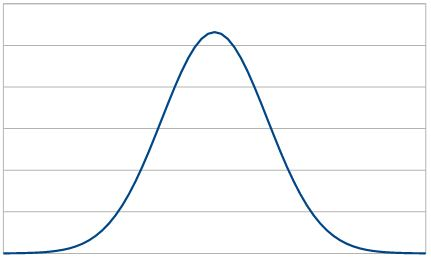# A Normal Distribution

How to make a normal curve from a spreadsheet; I had some help but this is how to do it in Libre Office. I can’t find the pages that helped me now, but others have written about this.

1. Create a scale in a column, in the example, it runs from -4 to +4. It has an average of 0.
2. Place the average of the scale and a standard deviation in named cells. In the example below, these are N_AVE (μ) and N_STDEV (σ). The standard deviation should be between the average and the scale limits.
3. From the range value, calculate the range scaled by the mean () * stddev ()
4. From the scaled range, use the normal distribution function to calculate the normal distribution value which can be charted.

This example shows one row in the table.

 Range Scale (Calc) n() value ```. row[RANGE] * σ + μ ``` ```=NORM.DIST( row[Scale (Calc)], μ,σ,false())```An example spreadsheet showing a Normal Distribution

This site uses Akismet to reduce spam. Learn how your comment data is processed.